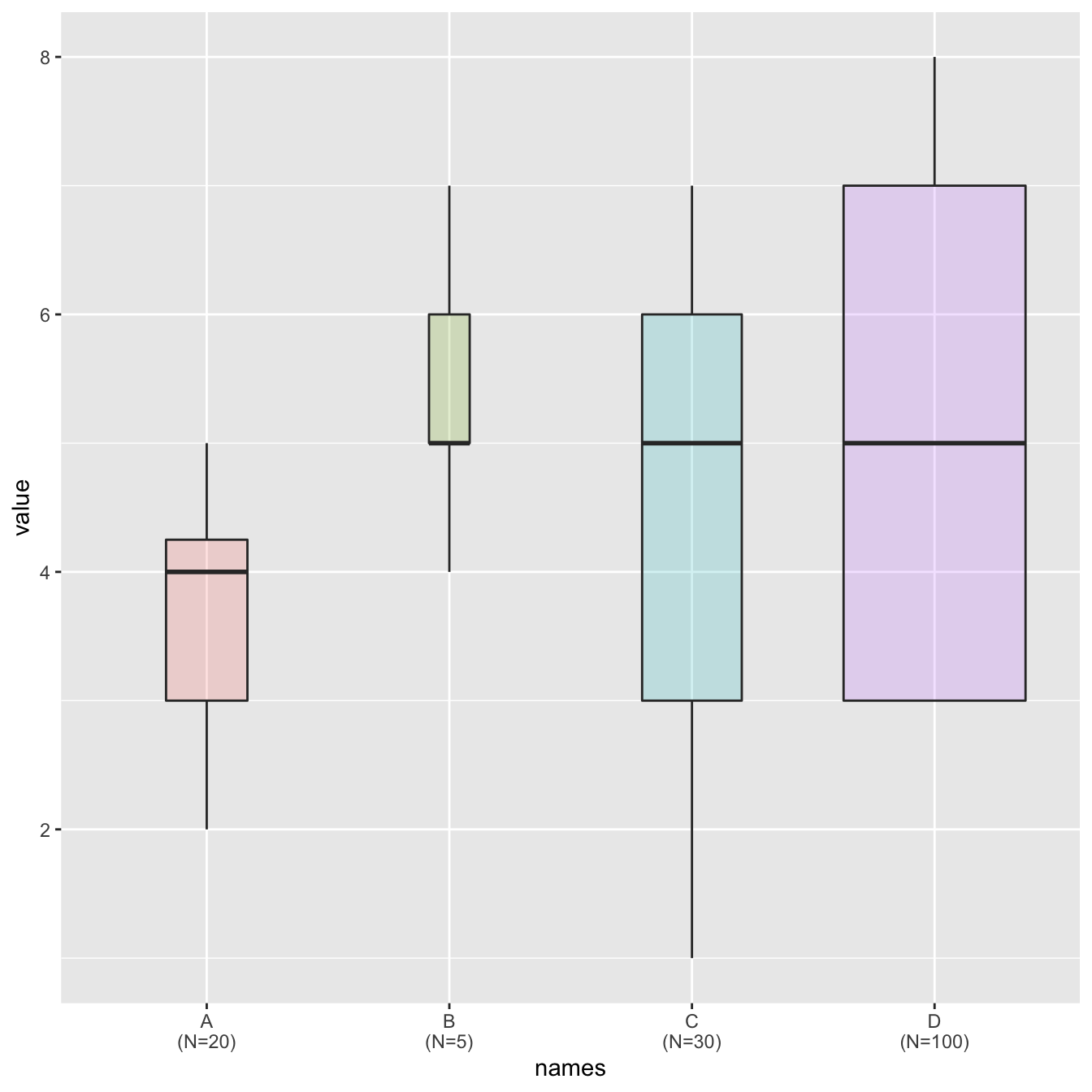# Ggplot2 boxplot with variable width

Boxplots hide the category sample sizes. One way to tackle this issue is to build boxplot with width proportionnal to sample size. Here is how to do it with R and ggplot2.

# Grouped boxplot

Boxplot are often critized for hiding the underlying distribution of each category. Since individual data points are hidden, it is also impossible to know what sample size is available for each category.

In this example, box widths are proportional to sample size thanks to the `varwidth` option. On top of that, the exact sample size is added to the X axis labels for more accuracy.``````# library
library(ggplot2)

# create data
names <- c(rep("A", 20) , rep("B", 5) , rep("C", 30), rep("D", 100))
value <- c( sample(2:5, 20 , replace=T) , sample(4:10, 5 , replace=T), sample(1:7, 30 , replace=T), sample(3:8, 100 , replace=T) )
data <- data.frame(names,value)

# prepare a special xlab with the number of obs for each group
my_xlab <- paste(levels(data\$names),"\n(N=",table(data\$names),")",sep="")

# plot
ggplot(data, aes(x=names, y=value, fill=names)) +
geom_boxplot(varwidth = TRUE, alpha=0.2) +
theme(legend.position="none") +
scale_x_discrete(labels=my_xlab)``````

Related chart types

## Contact

This document is a work by Yan Holtz. Any feedback is highly encouraged. You can fill an issue on Github, drop me a message on Twitter, or send an email pasting yan.holtz.data with gmail.com.